Next: Using the monotonicity Up: General purpose solving algorithm Previous: General purpose solving algorithm   Contents

Mathematical background

Assume now that we are able to compute the jacobian matrix of the system of functions. We will use this jacobian for improving the evaluation of the function intervals using two approaches:

1. use the monotonicity of the function
2. use the gradient for the evaluation of the function intervals
Note also that this method may be used to solve a system composed of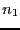equations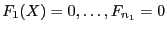,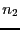inequalities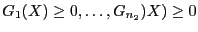and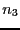inequalities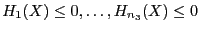. Note also that not all the function must be differentiable to use this procedure: only one of them is sufficient. In that case you will have however to set special values in the gradient function (see 2.4.2.2).

A notable difference with the previous procedure is that we use Moore theorem (see section 3.1.1) to determine if a unique solution exists in a given box, in which case we use Krawczyk method for determining this solution (see section 2.10). Therefore if the algorithm proposes as solution a point instead of a range this imply that this solution has been obtained as the result of Moore theorem. Note however that getting a range for a solution instead of a point does not always imply that we have a singular solution. For example it may happen that the solution is exactly at one extremity of a box (see example in section 2.4.3.2) which a case that our algorithm does not handle very well. A local analysis of the solution should however confirm quickly if the solution is indeed singular.

In addition we use also the inflation method presented in section 3.1.6 to increase the width of the box in which we may guarantee that there is a unique solution.

Hence this algorithm allows to determine exact solutions in the sense that we determine boxes that contains a unique solution and provides a guaranteed numerical scheme that allows for the calculation of the solution.

In the same way we use the Hansen-Sengupta version of the interval Newton method to improve the boxes (see ). Note that an improved interval Newton that may benefit from the structure of the system is available (see section 3.1.4).

This algorithms allows also to determines the solutions of non-0 dimensional system, see section 2.2.

SubsectionsNext: Using the monotonicity Up: General purpose solving algorithm Previous: General purpose solving algorithm   Contents
Jean-Pierre Merlet 2012-12-20